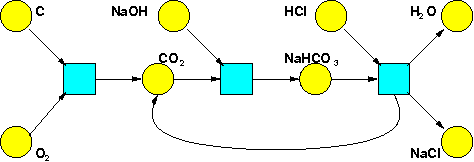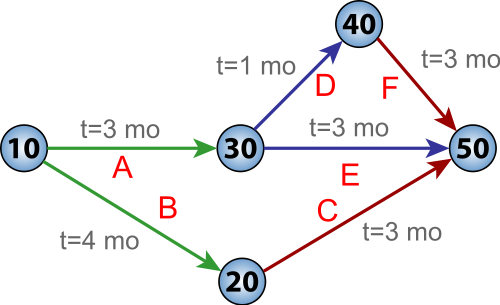Before we dive into the math of resource theories, let me say a bit more about what they're good for! I said they're good for answering questions like these: 1. Given what I have, _is it possible_ to get what I want? 2. Given what I have, _how much will it cost_ to get what I want? 3. Given what I have, _how long will it take_ to get what I want? 4. Given what I have, _what is the set of ways_ to get what I want? and also others. But let's see examples! **Chemistry.** Suppose we have a bunch of chemicals, and reactions involving these chemicals. Then we can ask which collections of molecules can turn into which other collections. We can also ask what's the set of ways in which this can happen. **Puzzle 55.** For example, suppose you have these chemical reactions: $\text{C} + \text{O}_2 \longrightarrow \text{CO}_2$ $\text{CO}_2 + \text{NaOH} \longrightarrow \text{NaHCO}_3$ $\text{NaHCO}_3 + \text{HCl} \longrightarrow \text{H}_2 \text{O} + \text{NaCl} + \text{CO}_2$ Can you use these to turn $\text{C} + \text{O}_2 + \text{NaOH} + \text{HCl}$ into $\text{CO}_2 + \text{H}_2\text{O} + \text{NaCl} \text{?}$ If so, how many ways can you do it? The "can you do it?" question here is called the **reachability problem**, and you can read about it here: * John Baez and Jacob Biamonte, _[Quantum Techniques for Stochastic Mechanics](https://arxiv.org/abs/1209.3632)_, Section 25.1: The Reachability Problem, World Scientific Press, 2018. If you like hard problems, the reachability problem is for you! The puzzle above is not hard, but in general the reachability problem is very hard. It was proved in 1981 that there's an algorithm to solve it, no matter what chemicals and reactions you choose. In other words, it's ["decidable"](https://en.wikipedia.org/wiki/Decidability_(logic)). However, it's known that no algorithm can solve it in [polynomial time](https://en.wikipedia.org/wiki/Time_complexity#Polynomial_time). The only known _upper bound_ on the runtime of an algorithm that solves the reachability problem is insanely bad... in fact, so bad that I'll make you read our book to learn how bad! So, there's a very interesting set of puzzles here for people skilled in [computational complexity theory](https://en.wikipedia.org/wiki/Computational_complexity_theory). One way to draw a bunch of chemicals and reactions involving them is a ["Petri net"](https://en.wikipedia.org/wiki/Petri_net). Here's the Petri net for the reactions I just listed:My book with Jacob is really about Petri nets. They're actually used in computer science more than chemistry! That's because they're a good way to draw a bunch of processes that take certain inputs and produce a bunch of outputs. Whenever we have this sort of situation, the reachability problem becomes important. **Scheduling.** Suppose you have a bunch of jobs to do, that take various amounts of time. And suppose you can only start some jobs when others are done. How long will it take to do all these jobs? This a hugely important problem, for example in big companies. One approach to solving it uses ["PERT charts"](https://en.wikipedia.org/wiki/Program_evaluation_and_review_technique), where "PERT" - businesspeople love acronyms but I hate them - stands for "program evaluation and review technique". Here's an example: